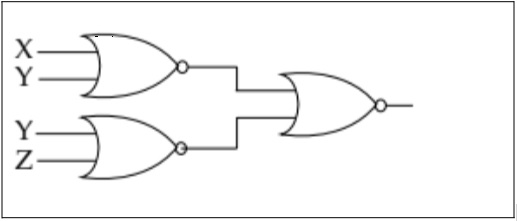MCQ questions (1 mark each)

Computer Science - Class 11
Chapter 12 Class 11 - Boolean Algebra

## (d) (X.Y)+(Y+Z)'((X+Y)’ + (Y+Z)’)’ =  ((X+Y)’)’ . ((Y+Z)’)’           (Using DeMorgan’s first law)

= (X+Y).(Y+Z)                       (Using Inversion law)

So, the correct answer is (b).

Learn in your speed, with individual attention - Teachoo Maths 1-on-1 Class